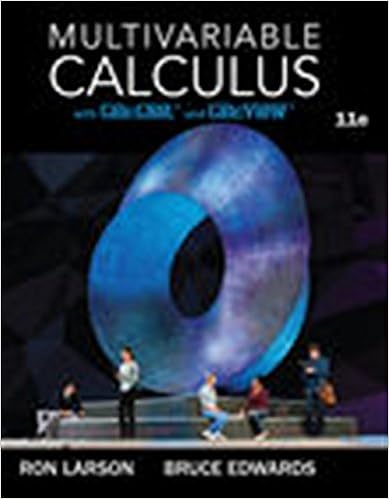# A the potential difference decreases and the stored

• Notes
• 373
• 100% (5) 5 out of 5 people found this document helpful

This preview shows page 243 - 246 out of 373 pages.

##### We have textbook solutions for you!
The document you are viewing contains questions related to this textbook.The document you are viewing contains questions related to this textbook.
Chapter 12 / Exercise 46
Multivariable Calculus
LarsonExpert Verified
(A)The potential difference decreases and the stored energy increases(B)Both the potential difference and the stored energy increase(C)The potential difference increases and the stored energy decreases(D)Both the potential difference and the stored energy decrease(E)Both the potential difference and the stored energy remain the sameExplanations1. AOhm’s Law tells us that current and voltage are directly proportional: doubling the voltage will also double the current.2. DThe power dissipated in a resistor is given by the formula P= V2/R. Since P and R are inversely proportional, multiplying the resistance by four will divide the power by four.3. EThe resistance for a wire is given by the formula R = L/A, where is the resistivity of the material the wire is made of, L is the length of the wire, and A is the cross-sectional area of the wire.The value of varies from material to material, so the material the wire is made of does affect the resistance in the wire, which is why we don’t wire our houses with glass or wooden wires. The length of the wire, L, also affects the resistance, since the longer a wire gets, the farther the electrons in the wire have to travel. The cross-sectional area, A, and hence the diameter of the wire affects the resistance, since charges have more room to move in a wider wire. Since all three of the statements are true, the answer is E.4. BAccording to Ohm’s Law, V = IR: current is directly proportional to potential difference. If the potential difference across is half the potential difference across , and if and have the same resistance, then the current through is half the current through .5. B243
##### We have textbook solutions for you!
The document you are viewing contains questions related to this textbook.The document you are viewing contains questions related to this textbook.
Chapter 12 / Exercise 46
Multivariable Calculus
LarsonExpert Verified
The equivalent resistance, , of two identical resistors in parallel is given by the formula:The equivalent resistance of three identical resistors in parallel is given by the formula:The ratio, then, between the new resistance and the old is:6. DAccording to Ohm’s Law, V = IR, current and resistance are inversely proportional. In the previous question, we saw that the new resistance is 2/3the old resistance. That means that, inversely, the new current is 3/2 times the old resistance.7. EThe power dissipated in a resistor is given by the formula P = I2R, which in this case has a value of 20 W. The heat dissipated in a resistor is given by the formula H = Pt: every second, the resistor dissipates 20 J of heat. Since we are looking at a 10-second period, the total heat dissipated is 200 J.8. AThe equivalent capacitance of two capacitors in series is:244
The equivalent capacitance of two capacitors in parallel is simply the sum of the two capacitors, so = + = 2C. The ratio between the equivalent capacitance of the two capacitors in series and the two capacitors in parallel is therefore:9. CThe energy stored in a capacitor is . When a dielectric with a dielectric constant of is inserted between the plates of a capacitor with capacitance C, the new capacitance is C. So (C) is the correct answer.
•••